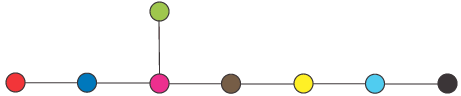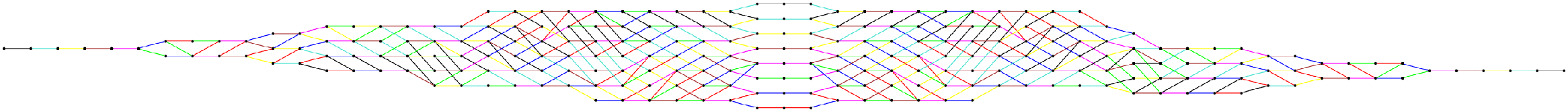# Dynkin Diagram and Crystal Graph for E8This is the Dynkin Diagram of E8. See what is E8 for a description of the E8 root system. This is a set of roots in R8. The 8 nodes of the Dynkin diagram correspond to 8 roots of the E8 root system, which are a basis of the vector space.This is a picture of the 248-dimensional Lie algebra of E8. This Lie algebra is a complex vector space, of dimension 248. It is equipped with a Lie bracket operator: for X,Y in the Lie algebra, so is [X,Y]. There are 248 nodes in the picture, one for each basis element of the Lie algebra. Label the nodes 1,...,248, and the corresponding elements of the Lie algebra Z1,...,Z248.

The Lie algebra E8 is generated by 16 elements Xred, Yred, Xblue, Yblue,..., Xblack, Yblack corresponding to the nodes in the Dynkin diagram. These are elements of the Lie algebra, and every element of the Lie algebra can be otained by taking brackets [,] of these.

The Lie algebra of E8 is generated by 8 pairs of elements (X,Y), one pair for each of the colored nodes in the Dynkin diagram. Suppose a node in the big diagram does not have a red edge, for example. This means the operators X and Y take Zi to a multiple of itself. If node i is connected to node j by a red edge, then X and Y take Zi to some linear combination of Zi and Zj.